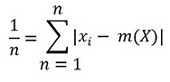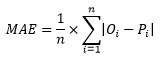#Mean Absolute Error & Mean Absoulte Deviation Calculation#MAD, MAE Calculator

Instantly calculates the mean absolute error & mean absoulte deviation.### Mean Absoulte Deviation (MAD) Formula

Mean absolute deviation of a component of a data set is the total difference between that component and a given point.This calculator uses the above formula for calculating the mean absolute deviation

### Mean Absoulte Error (MAE) Formula

It is a quantity used to measure how close the predicted values are to the practical outcomes.Where,

|Oi - Pi| = the absolute errors

σ = summation symbol